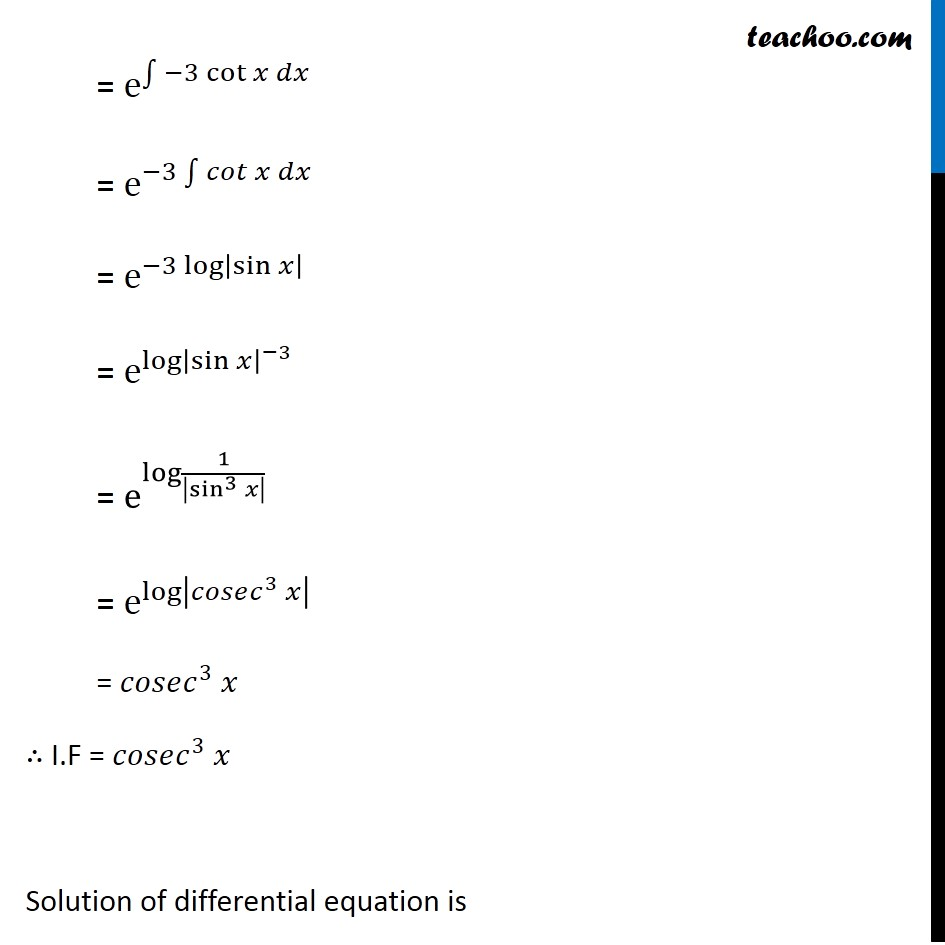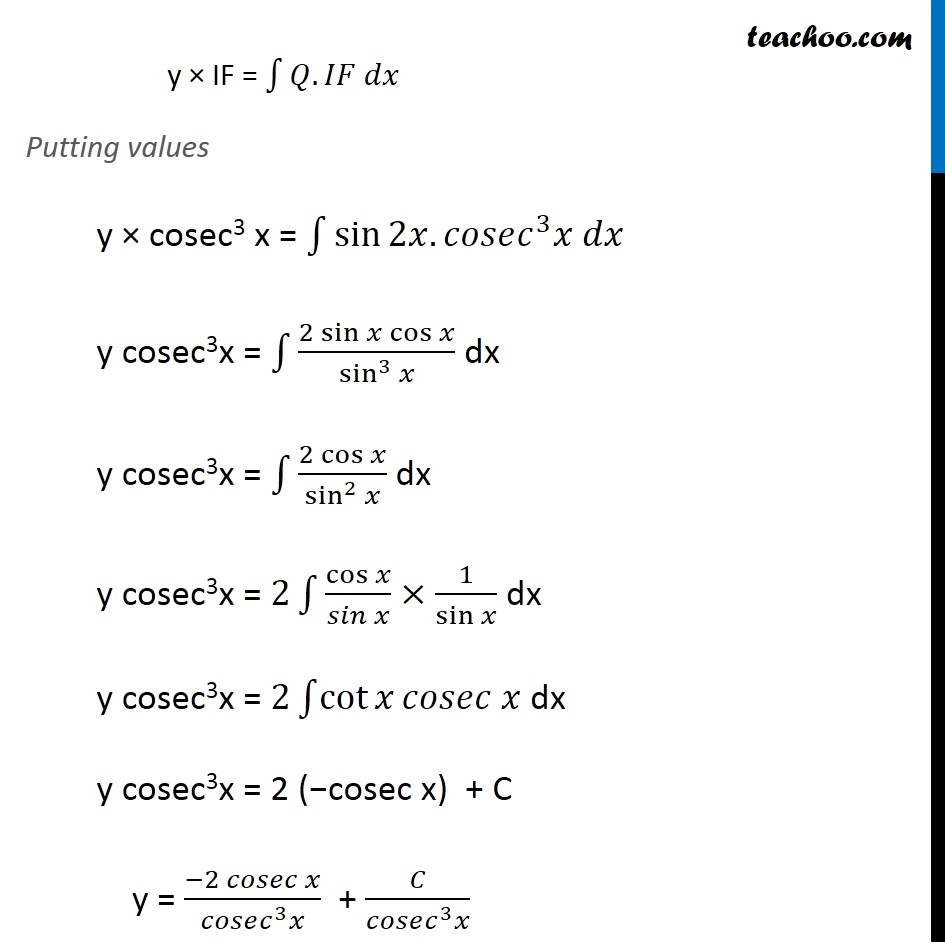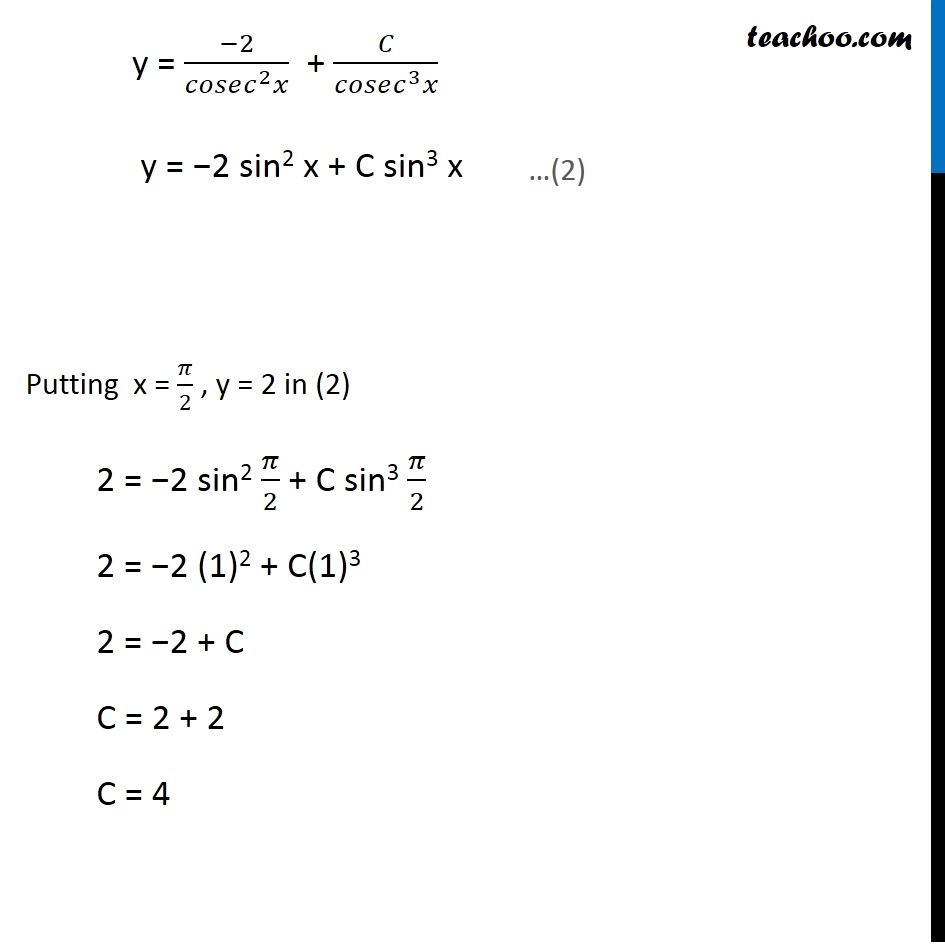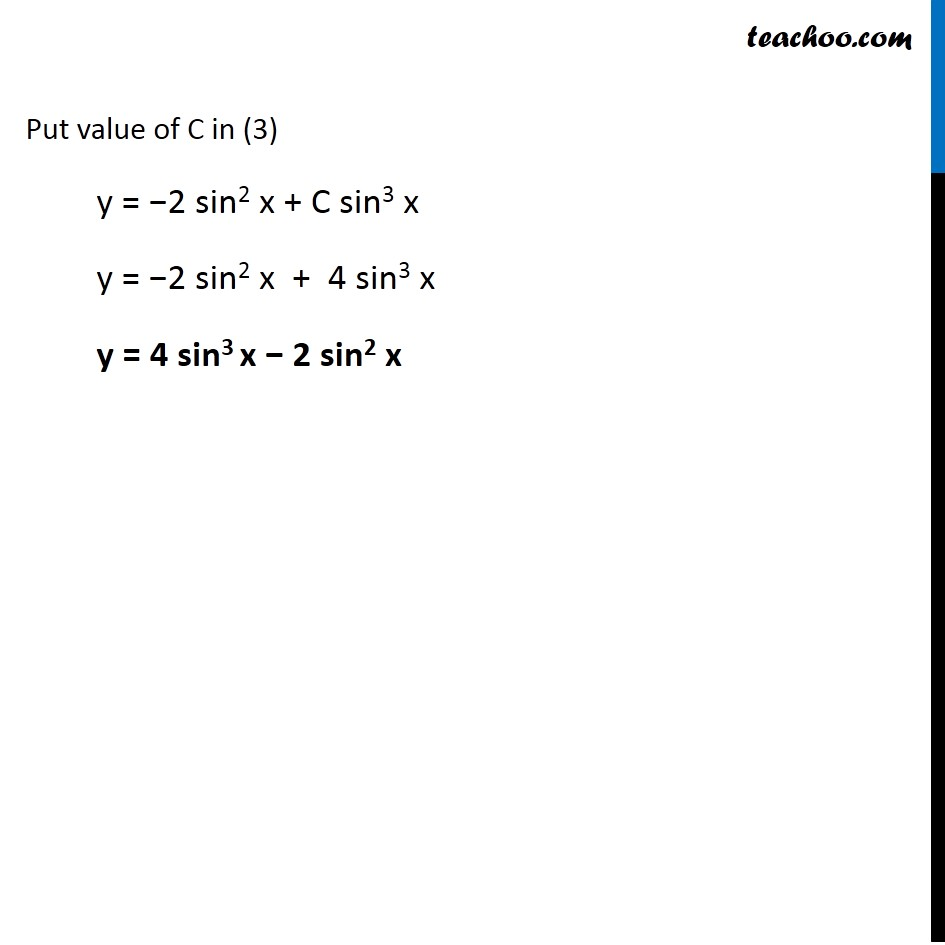1. Chapter 9 Class 12 Differential Equations (Term 2)
2. Serial order wise
3. Ex 9.6

Transcript

Ex 9.6, 15 For each of the differential equations given in Exercises 13 to 15 , find a particular solution satisfy the given condition : 𝑑𝑦/𝑑𝑥−3𝑦 cot⁡〖𝑥=sin⁡〖2𝑥;𝑦=2〗 〗 when 𝑥= 𝜋/2 𝑑𝑦/𝑑𝑥−3𝑦 cot⁡〖𝑥=sin⁡2𝑥 〗 𝑑𝑦/𝑑𝑥 + (−3 cot x) y = (sin 2x) Comparing with 𝑑𝑦/𝑑𝑥 + Py = Q P = −3 cot x & Q = sin 2x Finding Integrating factor (IF) IF = e^∫1▒𝑝𝑑𝑥 …(1) = e^∫1▒〖−3 cot⁡〖𝑥 𝑑𝑥〗 〗 = e^(−3∫1▒〖𝑐𝑜𝑡 𝑥 𝑑𝑥〗) = e^(−3 log⁡|sin⁡𝑥 | ) = e^log⁡〖|sin⁡𝑥 |^(−3) 〗 = e^log⁡〖1/|sin^3⁡𝑥 | 〗 = e^log⁡|𝑐𝑜𝑠𝑒𝑐^3 𝑥| = 𝑐𝑜𝑠𝑒𝑐^3 𝑥 ∴ I.F = 𝑐𝑜𝑠𝑒𝑐^3 𝑥 Solution of differential equation is y × IF = ∫1▒〖𝑄.𝐼𝐹 𝑑𝑥〗 Putting values y × cosec3 x = ∫1▒sin⁡〖2𝑥. 𝑐𝑜𝑠𝑒𝑐^3 𝑥 𝑑𝑥〗 y cosec3x = ∫1▒(2 sin⁡〖𝑥 cos⁡𝑥 〗)/sin^3⁡𝑥 dx y cosec3x = ∫1▒(2 cos⁡𝑥)/sin^2⁡𝑥 dx y cosec3x = 2∫1▒〖cos⁡𝑥/𝑠𝑖𝑛⁡𝑥 ×1/sin⁡𝑥 〗 dx y cosec3x = 2∫1▒〖cot⁡𝑥 𝑐𝑜𝑠𝑒𝑐 𝑥〗 dx y cosec3x = 2 (−cosec x) + C y = (−2 𝑐𝑜𝑠𝑒𝑐 𝑥)/(𝑐𝑜𝑠𝑒𝑐^3 𝑥) + 𝐶/(𝑐𝑜𝑠𝑒𝑐^3 𝑥) y = (−2)/(𝑐𝑜𝑠𝑒𝑐^2 𝑥) + 𝐶/(𝑐𝑜𝑠𝑒𝑐^3 𝑥) y = −2 sin2 x + C sin3 x Putting x = 𝜋/2 , y = 2 in (2) 2 = −2 sin2 𝜋/2 + C sin3 𝜋/2 2 = −2 (1)2 + C(1)3 2 = −2 + C C = 2 + 2 C = 4 …(2) Put value of C in (3) y = −2 sin2 x + C sin3 x y = −2 sin2 x + 4 sin3 x y = 4 sin3 x − 2 sin2 x

Ex 9.6

Chapter 9 Class 12 Differential Equations (Term 2)
Serial order wise